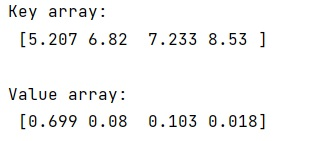# Dictionary keys and values to separate NumPy arrays

Learn, how to separate dictionary keys and values to create two separate NumPy arrays?
Submitted by Pranit Sharma, on March 01, 2023

NumPy is an abbreviated form of Numerical Python. It is used for different types of scientific operations in python. Numpy is a vast library in python which is used for almost every kind of scientific or mathematical operation. It is itself an array which is a collection of various methods and functions for processing the arrays.

## Separate the dictionary keys and values to create two separate NumPy arrays

Suppose that we are given a dictionary and we need to separate the keys and values into two numpy arrays.

For this purpose, we can use the numpy.formiter() two times for keys and values respectively. This function creates a new 1-dimensional array from an iterable object. Inside this, we can pass the dict.keys() and dict.values() separately.

Let us understand with the help of an example,

## Python code to separate dictionary keys and values to create two separate NumPy arrays

```# Import numpy
import numpy as np

# Creating a dictionary
d = {5.207: 0.699, 6.820: 0.080, 7.233: 0.103, 8.530: 0.018}

# Separating key and values in two arrays
key = np.fromiter(d.keys(), dtype=float)
vals = np.fromiter(d.values(), dtype=float)

# Display result
print("Key array:\n",key,"\n")
print("Value array:\n",vals,"\n")
```

Output:Languages: » C » C++ » C++ STL » Java » Data Structure » C#.Net » Android » Kotlin » SQL
Web Technologies: » PHP » Python » JavaScript » CSS » Ajax » Node.js » Web programming/HTML
Solved programs: » C » C++ » DS » Java » C#
Aptitude que. & ans.: » C » C++ » Java » DBMS
Interview que. & ans.: » C » Embedded C » Java » SEO » HR
CS Subjects: » CS Basics » O.S. » Networks » DBMS » Embedded Systems » Cloud Computing
» Machine learning » CS Organizations » Linux » DOS
More: » Articles » Puzzles » News/Updates